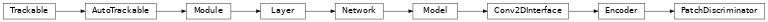# PatchDiscriminator¶

Inheritance Diagramclass ashpy.models.convolutional.discriminators.PatchDiscriminator(input_res, min_res, kernel_size, initial_filters, filters_cap, use_dropout=True, dropout_prob=0.3, non_linearity=<class 'tensorflow.python.keras.layers.advanced_activations.LeakyReLU'>, normalization_layer=<class 'ashpy.layers.instance_normalization.InstanceNormalization'>, use_attention=False)[source]

Pix2Pix discriminator.

The last layer is an image in which each pixels is the probability of being fake or real.

Examples

x = tf.ones((1, 64, 64, 3))

# instantiate the PathDiscriminator
patchDiscriminator = PatchDiscriminator(input_res=64,
min_res=16,
kernel_size=5,
initial_filters=64,
filters_cap=512,
)

# evaluate passing x
output = patchDiscriminator(x)

# the output shape is the same as the input shape
print(output.shape)

(1, 12, 12, 1)


Methods

 __init__(input_res, min_res, kernel_size, …) Patch Discriminator used by pix2pix. call(inputs[, training, return_features]) Forward pass of the PatchDiscriminator.

Attributes

 activity_regularizer Optional regularizer function for the output of this layer. dtype dynamic inbound_nodes Deprecated, do NOT use! Only for compatibility with external Keras. input Retrieves the input tensor(s) of a layer. input_mask Retrieves the input mask tensor(s) of a layer. input_shape Retrieves the input shape(s) of a layer. input_spec Gets the network’s input specs. layers losses Losses which are associated with this Layer. metrics Returns the model’s metrics added using compile, add_metric APIs. metrics_names Returns the model’s display labels for all outputs. name Returns the name of this module as passed or determined in the ctor. name_scope Returns a tf.name_scope instance for this class. non_trainable_variables non_trainable_weights outbound_nodes Deprecated, do NOT use! Only for compatibility with external Keras. output Retrieves the output tensor(s) of a layer. output_mask Retrieves the output mask tensor(s) of a layer. output_shape Retrieves the output shape(s) of a layer. run_eagerly Settable attribute indicating whether the model should run eagerly. sample_weights state_updates Returns the updates from all layers that are stateful. stateful submodules Sequence of all sub-modules. trainable trainable_variables Sequence of variables owned by this module and it’s submodules. trainable_weights updates variables Returns the list of all layer variables/weights. weights Returns the list of all layer variables/weights.
__init__(input_res, min_res, kernel_size, initial_filters, filters_cap, use_dropout=True, dropout_prob=0.3, non_linearity=<class 'tensorflow.python.keras.layers.advanced_activations.LeakyReLU'>, normalization_layer=<class 'ashpy.layers.instance_normalization.InstanceNormalization'>, use_attention=False)[source]

Patch Discriminator used by pix2pix.

When min_res=1 this is the same as a standard fully convolutional discriminator.

Parameters
• input_res (int) – Input Resolution.

• min_res (int) – Minimum Resolution reached by the discriminator.

• kernel_size (int) – Kernel Size used in Conv Layer.

• initial_filters (int) – number of filters in the first convolutional layer.

• filters_cap (int) – Maximum number of filters.

• use_dropout (bool) – whether to use dropout.

• dropout_prob (float) – probability of dropout.

• non_linearity (tf.keras.layers.Layer) – non linearity used in the model.

• normalization_layer (tf.keras.layers.Layer) – normalization layer used in the model.

• use_attention (bool) – whether to use attention.

_add_building_block(filters, use_bn=False)[source]

Construct the core of the tf.keras.Model.

The layers specified here get added to the tf.keras.Model multiple times consuming the hyper-parameters generated in the _get_layer_spec().

Parameters

filters (int) – Number of filters to use for this iteration of the Building Block.

_add_final_block(output_shape)[source]

Prepare the results of _add_building_block() for the final output.

Parameters

output_shape (int) – Amount of units of the last tf.keras.layers.Dense

call(inputs, training=False, return_features=False)[source]

Forward pass of the PatchDiscriminator.